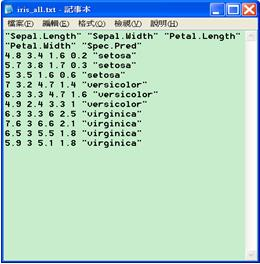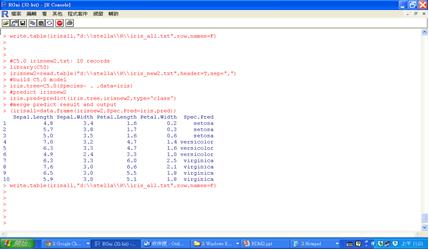﻿ R軟體資料探勘實務(上)--分類模型

# ntuccepaper2019

## 技術論壇

### 手機版選單

#### 字級

R軟體資料探勘實務(上)--分類模型
• 卷期：v0034
• 出版日期：2015-09-20

### 前言

1. 分類(Classification)：分類模型使用一個或多個輸入的值來預測一個或多個輸出目標的值。分類模型可幫助組織預測已知的結果，例如顧客是否購買、流失，腫瘤的判定是良性、惡性。
2. 關聯(Association)：關聯模型可找出資料間彼此的相關聯性，其中一個或多個實體與一個或多個其他實體相關聯。關聯模型在預測多個結果時非常有用，例如，購買了產品X的顧客也購買了產品Y和Z。
3. 集群(Clustering)：集群模型在不知道特定結果的情況下將數據劃分為具有類似輸入的記錄。在不知道特定結果的情況下，例如想將潛在用戶分成幾個相似的子群組，在客戶群中識別利益群體時，集群模型非常有用。

### 一. 建立分類模型的步驟

1. 讀入資料和資料準備

wdbc=na.exclude(wdbc)
wdbc=wdbc[,-1]
# test.index sampling 30% as testing group, remaining 70% as train group
n=0.3*nrow(wdbc)
test.index=sample(1:nrow(wdbc),n)
wdbc.train=wdbc[-test.index,]
wdbc.test=wdbc[test.index,]

2. 建立模型並輸出決策樹

install.packages("tree")
library(tree)
wdbc.tree=tree(diagnosis~.,data=wdbc.train)
wdbc.tree
summary(wdbc.tree)
plot(wdbc.tree)
text(wdbc.tree)

3. 結果分析

#train confusion matrix
diagnosis.train=wdbc\$diagnosis[-test.index]
train.pred=predict(wdbc.tree,newdata= wdbc.train, type='class')
(table.train=table(diagnosis.train,train.pred))
cat("Total records(train)=",nrow(wdbc.train), "\n")
cat("Correct Classification Ratio(train)=", sum(diag(table.train))/sum(table.train)*100,"%\n")
#test confusion matrix
diagnosis.test=wdbc\$diagnosis[test.index]
test.pred=predict(wdbc.tree,newdata=wdbc.test, type='class')
(table.test=table(diagnosis.test,test.pred))
cat("Total records(test)=",nrow(wdbc.test),"\n")
cat("Correct Classification Ratio(test)=", sum(diag(table.test))/sum(table.test)*100,"%\n")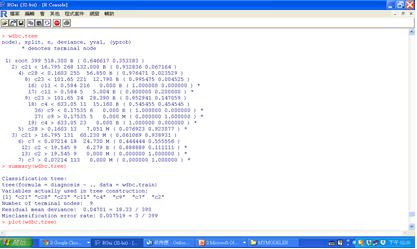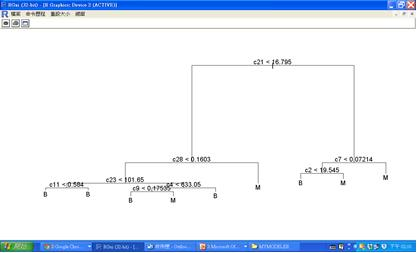### 二. R軟體常用的分類函數

1. C5.0決策樹(R提供C50套件的C5.0函數)
Quinlan在1986年所提出的ID3演算法後，因其無法處理連續屬性的問題且不適用在處理大的資料集，因此1993又發表C5.0的前身4.5，直到現在所使用的C5.0決策樹演算法。C5.0演算法的結果可產生決策樹及規則集兩種模型，並且依最大資訊增益的欄位來切割樣本，並重複進行切割直到樣本子集不能再被分割為止。C5.0能處理連續型變數與類別型的變數資料，目標欄位必須是類別型變數。這裡我們利用內建的iris資料集來建立模型。
install.packages("C50")
library(C50)
iris.C5=C5.0(Species~ . ,data=iris)
summary(iris.tree)
plot(iris.tree)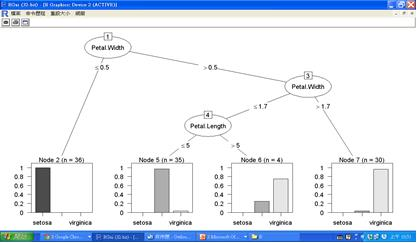2. CART分類迴歸樹(R提供tree套件的tree函數或rpart套件的rpart函數)

n=0.3*nrow(babies)
test.index=sample(1:nrow(babies),n)
babies.train=babies[-test.index,]
babies.test=babies[test.index,]
install.packages("rpart")
library(rpart)
babies.tree=rpart(bwt~gestation+parity+age+height+ weight+smoke, data= babies.train)
babies.tree
plot(babies.tree)
text(babies.tree)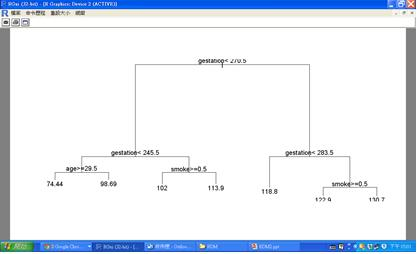bwt.train=babies\$bwt[-test.index]
train.pred=predict(babies.tree,newdata=babies.train,type="vector")
train.MAPE=mean(abs(bwt.train-train.pred)/bwt.train)
cat("MAPE(train)=",train.MAPE*100,"%\n")3. Random Forest隨機森林(R提供randomForest套件的randomForest函數)

install.packages("randomForest")
library(randomForest)
#clustering
(iris.clutrf=randomForest(iris[,-5]))
MDSplot(iris.clutrf,iris\$Species,palette=rep(1,3), pch= as.numeric(iris\$Species))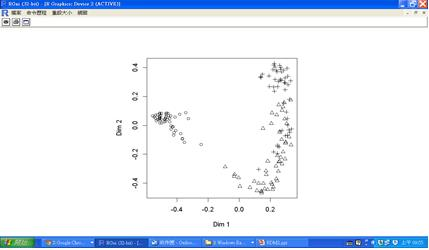### 三. 交叉驗證及模型部署

for ( i in 1:10)
{

}# irisnew2.txt: 10 records
library(C50)
#build C5.0 model
iris.tree=C5.0(Species~ . ,data=iris)
#predict irisnew2
iris.pred=predict(iris.tree,irisnew2,type='class')
#merge predict result and output
(irisall=data.frame(irisnew2,Spec.Pred=iris.pred))
write.table(irisall,"d:\\stella\\R\\iris_all.txt",row.names=F)